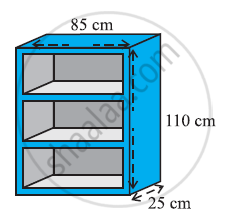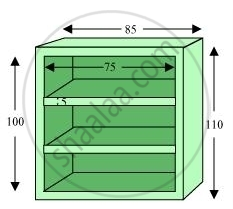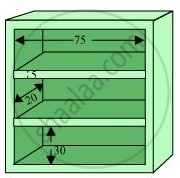# A wooden bookshelf has external dimensions as follows: Height = 110 cm, Depth = 25 cm, Breadth = 85 cm (see the given figure). The thickness of the plank is 5 cm everywhere. The external faces are to be polished and the inner faces are to be painted - Mathematics

A wooden bookshelf has external dimensions as follows: Height = 110 cm, Depth = 25 cm, Breadth = 85 cm (see the given figure). The thickness of the plank is 5 cm everywhere. The external faces are to be polished and the inner faces are to be painted. If the rate of polishing is 20 paise per cm2 and the rate of painting is 10 paise per cm2, find the total expenses required for polishing and painting the surface of the bookshelf.#### SolutionExternal height (l) of book self = 85 cm

External breadth (b) of book self = 25 cm

External height (h) of book self = 110 cm

External surface area of shelf while leaving out the front face of the shelf

= lh + 2 (lb bh)

= [85 × 110 + 2 (85 × 25 + 25 × 110)] cm2

= (9350 + 9750) cm2

= 19100 cm2

Area of front face = [85 × 110 − 75 × 100 + 2 (75 × 5)] cm2

= 1850 + 750 cm2

= 2600 cm2

Area to be polished = (19100 + 2600) cm= 21700 cm2

Cost of polishing 1 cm2 area = Rs 0.20

Cost of polishing 21700 cm2 area Rs (21700 × 0.20) = Rs 4340It can be observed that length (l), breadth (b), and height (h) of each row of the book shelf is 75 cm, 20 cm, and 30 cm respectively.

Area to be painted in 1 row = 2 (hb + lh

= [2 (75 + 30) × 20 + 75 × 30] cm2

= (4200 + 2250) cm2

= 6450 cm2

Area to be painted in 3 rows = (3 × 6450) cm= 19350 cm2

Cost of painting 1 cm2 area = Rs 0.10

Cost of painting 19350 cm2 area = Rs (19350 × 0.1)

= Rs 1935

Total expense required for polishing and painting = Rs (4340 + 1935)

= Rs 6275

Therefore, it will cost Rs 6275 for polishing and painting the surface of the bookshelf.

Concept: Volume of a Sphere
Is there an error in this question or solution?
Chapter 13: Surface Area and Volumes - Exercise 13.9 [Page 236]

#### APPEARS IN

NCERT Class 9 Maths
Chapter 13 Surface Area and Volumes
Exercise 13.9 | Q 1 | Page 236

Share